# Limits FGuilbert ORRHS Two Basic IDEAS of Calculus

• Slides: 24
Download presentation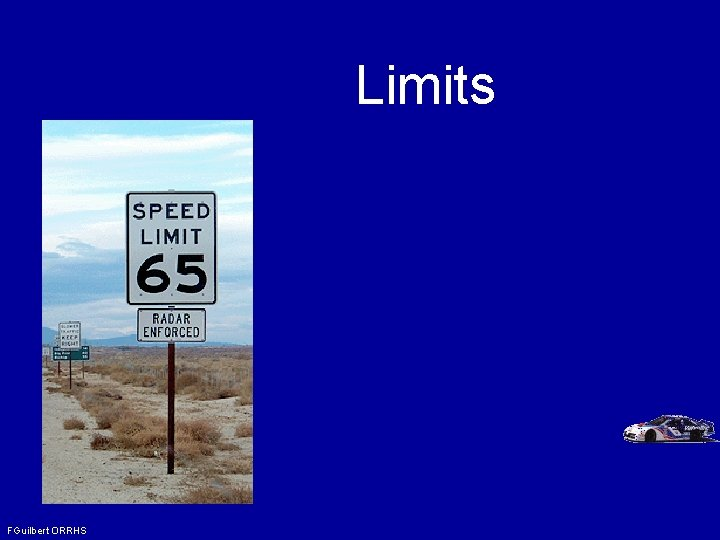Limits FGuilbert ORRHS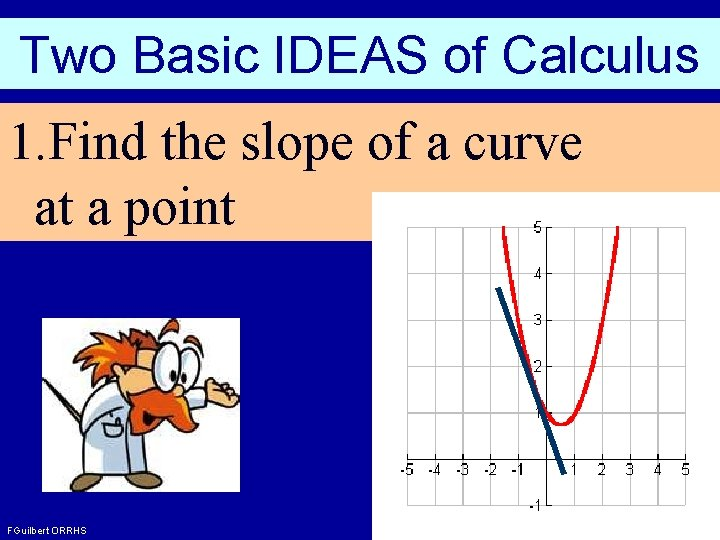Two Basic IDEAS of Calculus 1. Find the slope of a curve at a point FGuilbert ORRHS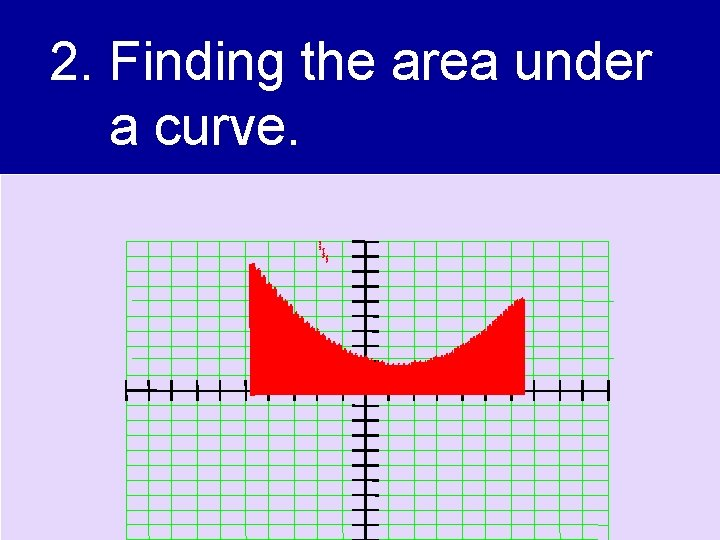2. Finding the area under a curve. FGuilbert ORRHSYou need to know about limits before you study those ideas. FGuilbert ORRHS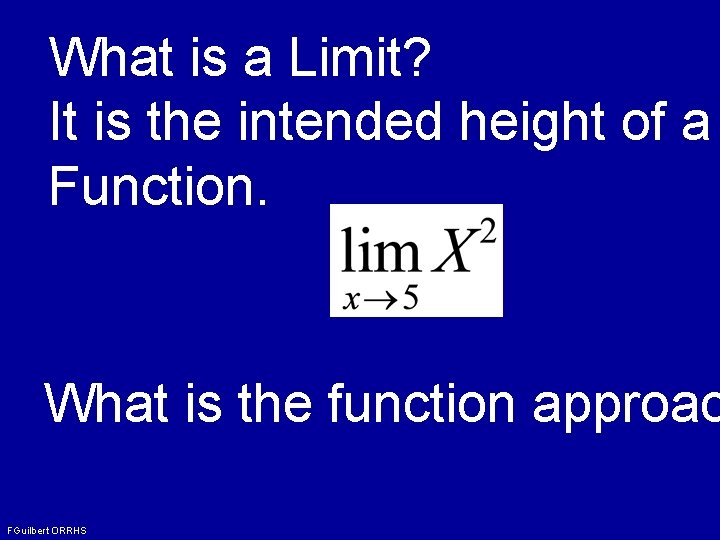What is a Limit? It is the intended height of a Function. What is the function approac FGuilbert ORRHSHow do you determine a Lim Numerically Graphically Substitution Definition Let’s look at some examples FGuilbert ORRHSAs the number of sides increases, the polygon gets closer to becoming a circle! FGuilbert ORRHS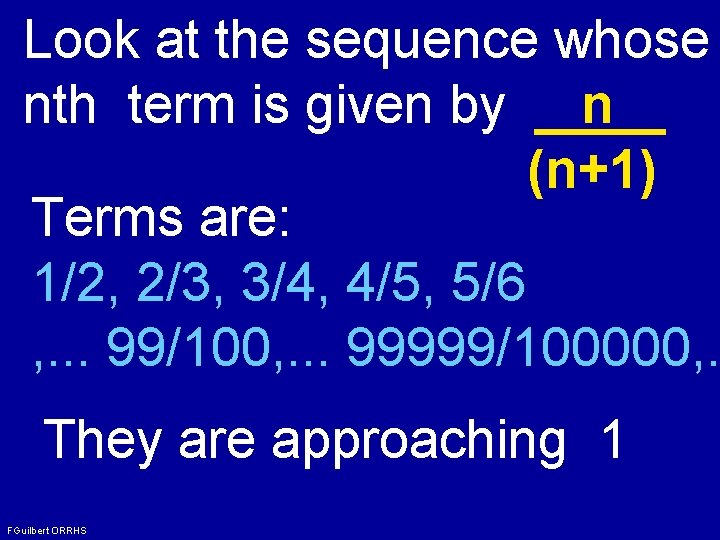Look at the sequence whose nth term is given by n (n+1) Terms are: 1/2, 2/3, 3/4, 4/5, 5/6 , . . . 99/100, . . . 99999/100000, . They are approaching 1 FGuilbert ORRHS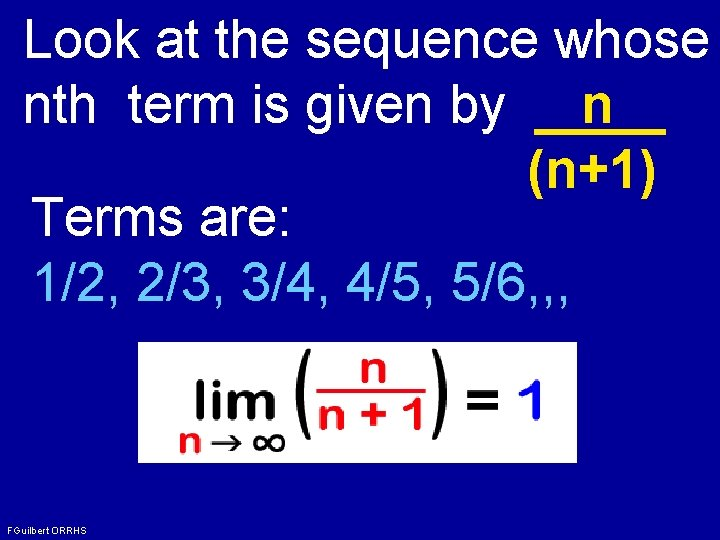Look at the sequence whose nth term is given by n (n+1) Terms are: 1/2, 2/3, 3/4, 4/5, 5/6, , , FGuilbert ORRHS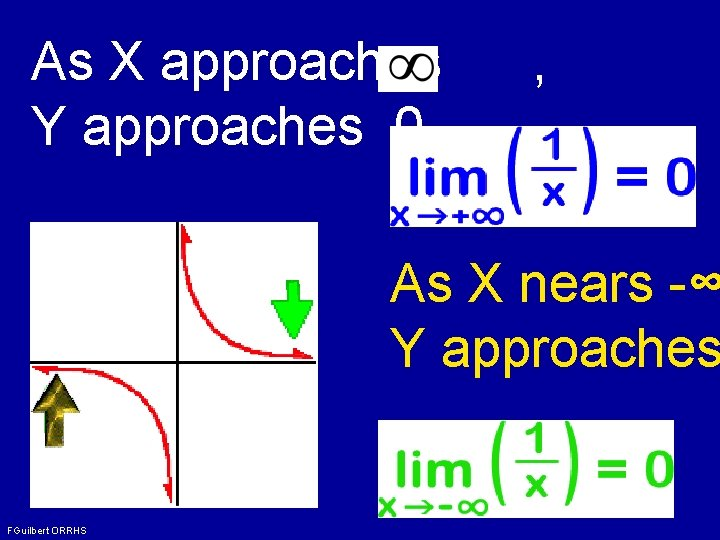As X approaches Y approaches 0. , As X nears -∞ Y approaches FGuilbert ORRHS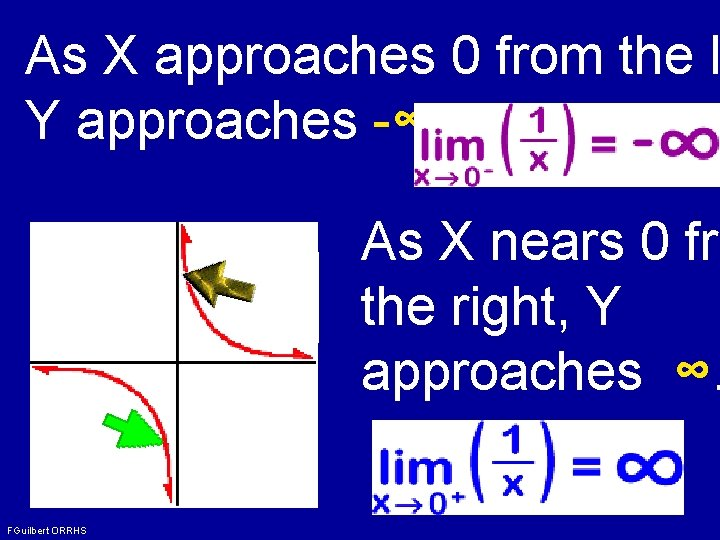As X approaches 0 from the l Y approaches -∞. As X nears 0 fro the right, Y approaches ∞. FGuilbert ORRHSLimits do NOT Agree FGuilbert ORRHS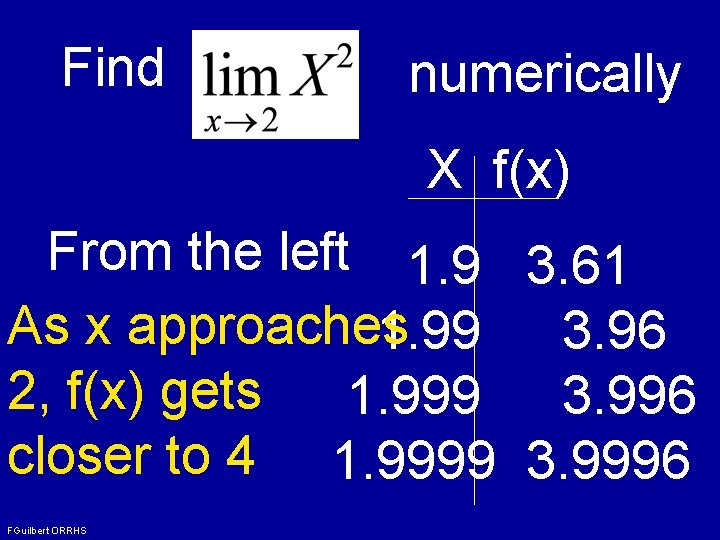Find numerically X f(x) From the left 1. 9 3. 61 As x approaches 1. 99 3. 96 2, f(x) gets 1. 999 3. 996 closer to 4 1. 9999 3. 9996 FGuilbert ORRHS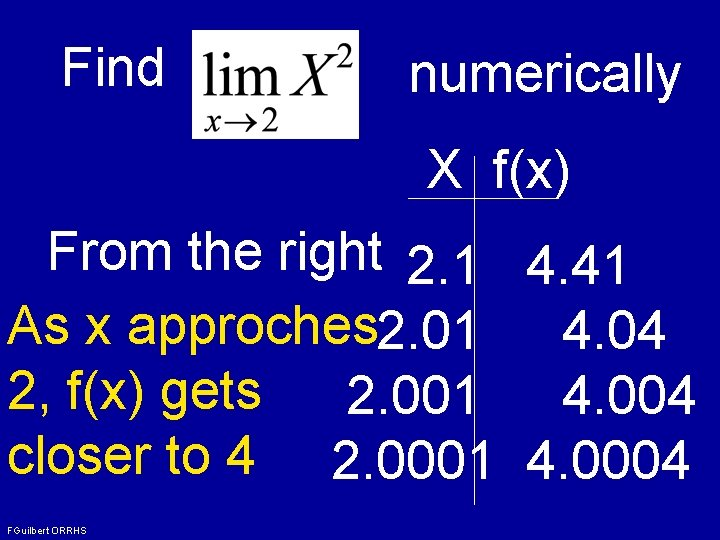Find numerically X f(x) From the right 2. 1 4. 41 As x approches 2. 01 4. 04 2, f(x) gets 2. 001 4. 004 closer to 4 2. 0001 4. 0004 FGuilbert ORRHS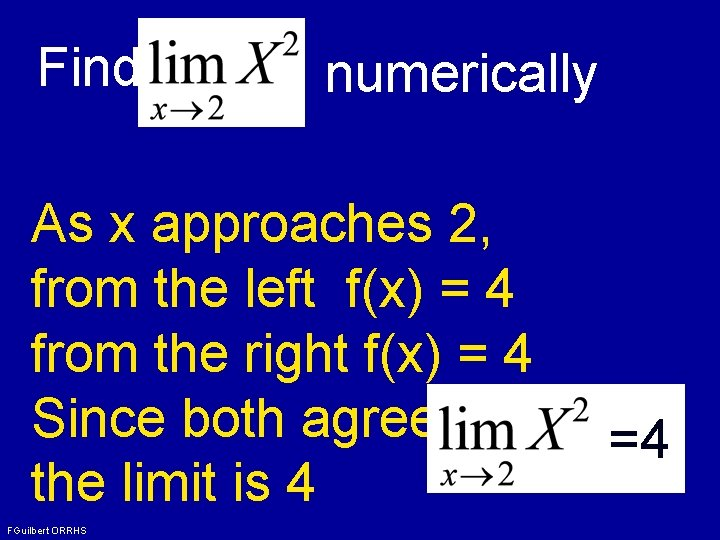Find numerically As x approaches 2, from the left f(x) = 4 from the right f(x) = 4 Since both agree, the limit is 4 FGuilbert ORRHS =4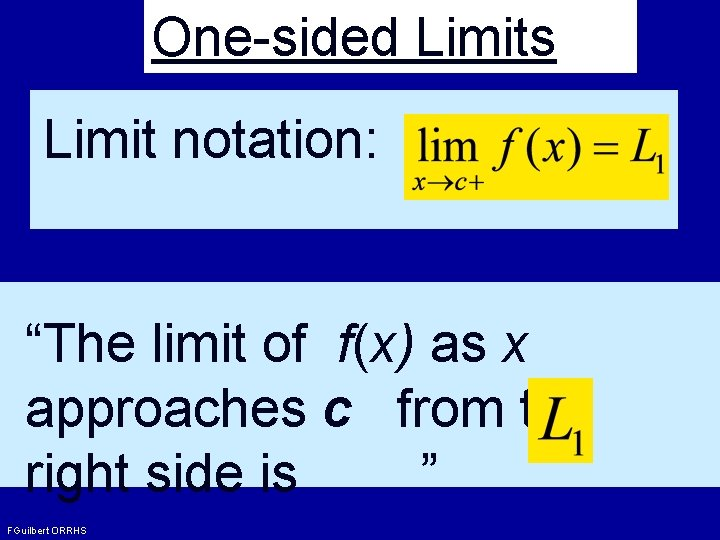One-sided Limits Limit notation: “The limit of f(x) as x approaches c from the right side is ” FGuilbert ORRHS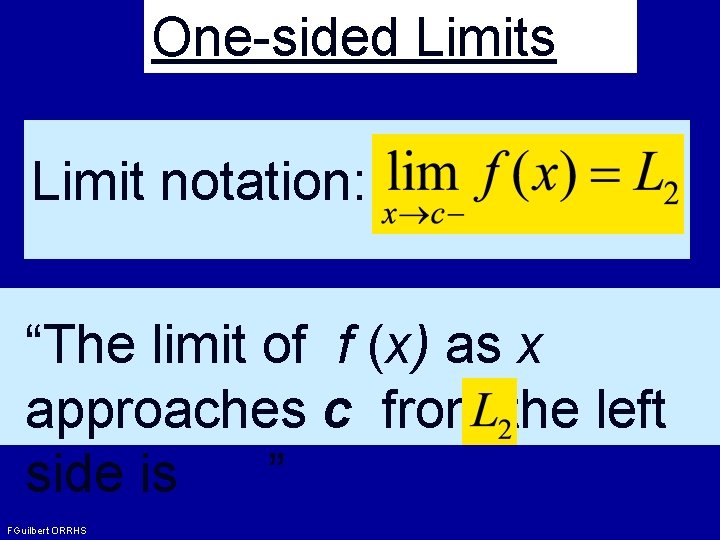One-sided Limits Limit notation: “The limit of f (x) as x approaches c from the left side is ” FGuilbert ORRHS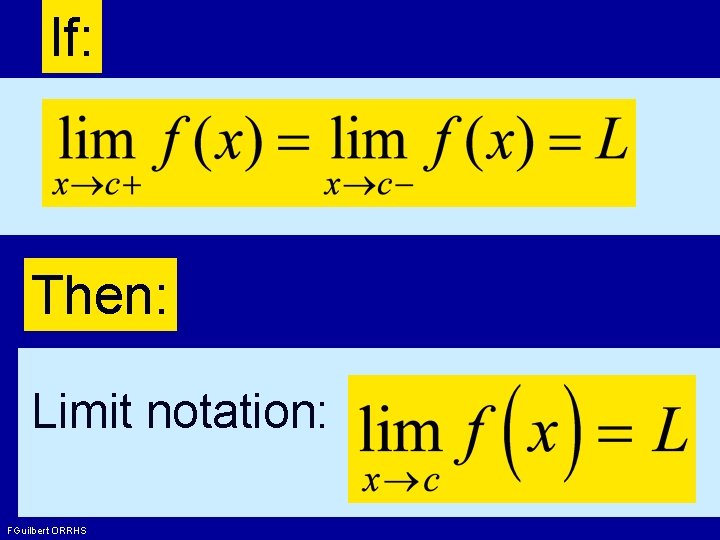If: Then: Limit notation: FGuilbert ORRHS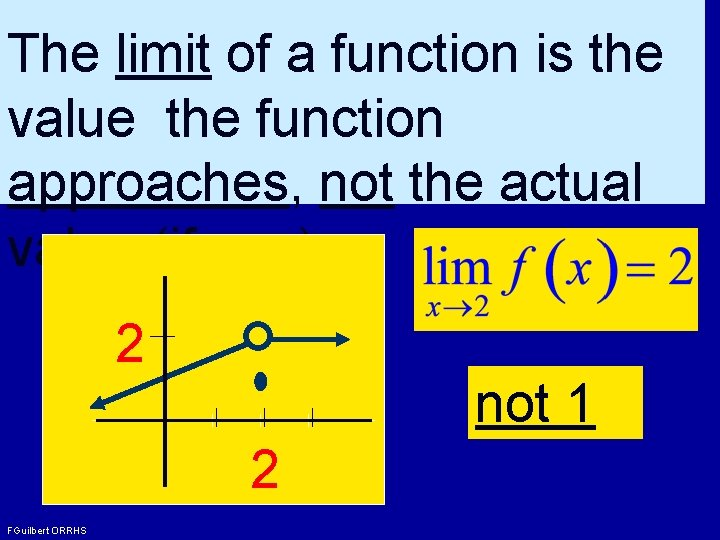The limit of a function is the value the function approaches, not the actual value (if any). 2 not 1 2 FGuilbert ORRHS2 1 1 2 3 4 At x=1: Does n exist. Left and R Limits are not = Left limit Right limi FGuilbert ORRHS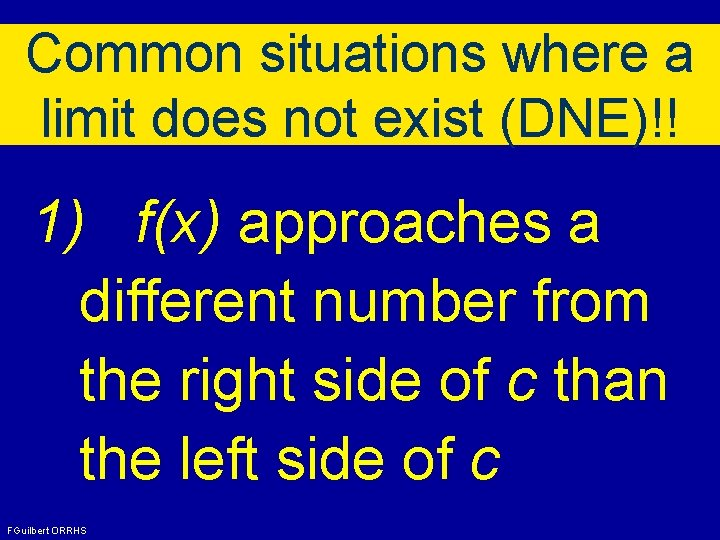Common situations where a limit does not exist (DNE)!! 1) f(x) approaches a different number from the right side of c than the left side of c FGuilbert ORRHSCommon situations where a limit does not exist (DNE)!! 2) f(x) increases or decreases without bound as x approaches c. FGuilbert ORRHS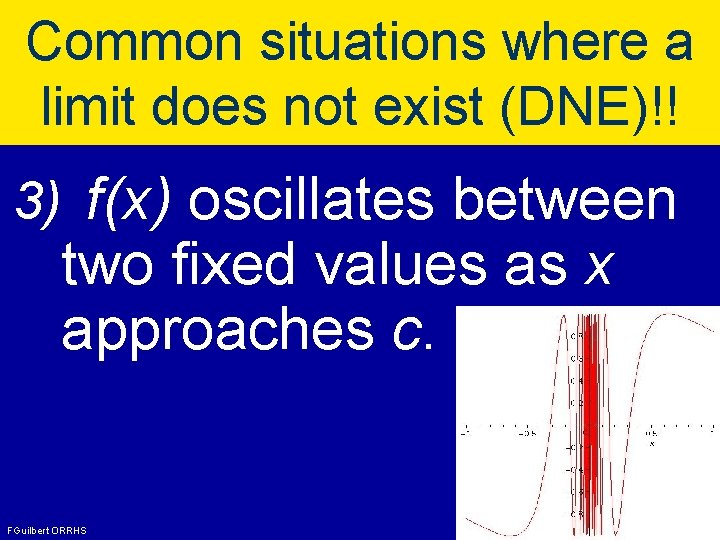Common situations where a limit does not exist (DNE)!! 3) f(x) oscillates between two fixed values as x approaches c. FGuilbert ORRHSOnce we accept our limit we can go beyond them FGuilbert ORRHS